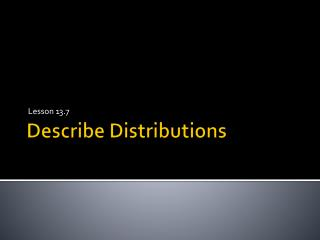DownloadDownload PresentationDescribe Distributions

# Describe Distributions

Télécharger la présentation## Describe Distributions

- - - - - - - - - - - - - - - - - - - - - - - - - - - E N D - - - - - - - - - - - - - - - - - - - - - - - - - - -
##### Presentation Transcript

1. Lesson 13.7 Describe Distributions

2. Vocabulary –Unit 13 • peak • symmetry • gap • cluster • lower quartile • upper quartile • box plot • mean absolute deviation • measure of variability • range • interquartile range • distribution

3. Objectives - 13.7 Describe Distributions • Content Objective: IWBAT to describe the distribution of a data set collected to answer a statistical question. • Language Objective: IWBAT write and explain to my partner how to describe the distribution of a data set collected to answer a statistical question.

4. Essential Question • How can you describe the distribution of a data set collected to answer a statistical question?

5. Problem of the Day • The ages of people in a karate class are 19, 24, 28, 20, 19, 21, and 27. What is the range of ages? • a. 8 • b. 9 • c. 19 • d. 28

6. Data Set Practice • 7,8,8,14,12,8,7,16,16,8,7,15,14,16,12,8,8,8,13, and 15 years. • Set up axes on grid paper. • “Age” on the horizontal axis. • “Frequency” on the vertical axis. • Create a dot plot